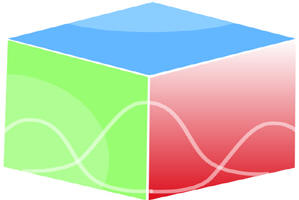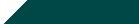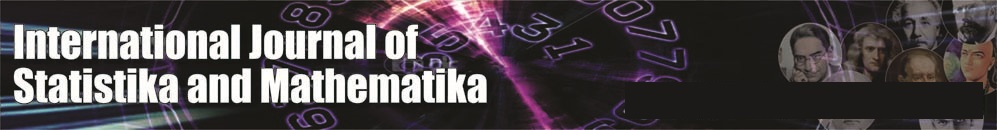``Home| Journals | Statistics Online Expert | About Us | Contact UsUntitled Document

[

A New Topology τ*g via g-local Functions in Ideal Topological Spaces

Pauline Mary Helen

Associate Professor, Nirmala College, Coimbatore, Tamil Nadu, INDIA.

[email protected]

Research Article

Abstract: In this paper we introduce and study three different notions via ideals namely g-local function, the set operator Ψg and g-compatibility of τ with I. We characterize these new sorts. Several properties of them have been studied and their relationships with other types of similar operators are also investigated.

Keywords:*g-additive space, *g- finitely additive space,g-local function,(  ) *g operator, Ψg operator,g-compatibility of τ with I

1. Introduction

Ideals topological spaces have been first introduced by K. Kuratowski in 1930.Vaidyanathaswamy  introduced local function in 1945 and defined a topology τ. In this paper we introduce and study three different notions via ideals namely  g-local function, the set operator Ψg , and g-compatibility of τ with I and investigate their relationships with other types of similar operators .

2. Preliminaries

Let  be a topological space and . We denote closure of A and interior of A by

and   respectively.

Definition 2.1: A set A in a topological space (X, t) is said to be a generalized closed set (briefly g-closed) if cl(A) ÍU whenever A ÍU and U is open in (X, t).

The class of g - open set in X will be denoted by  GO(X,t).

Definition.2.3 An ideal   on a non empty set  is a collection of subsets of  which satisfies the following properties:(i) ,         (ii) ,     . A topological space  with an ideal  on  is called an ideal topological space and is denoted by . Let be a subset of .  is an ideal on  and by  we denote the ideal topological subspace. Let  be the power set of , then a set operator (  )*:  called the local function  of A with respect to  and  is defined as follows:For ,  for every open set  containing  We simply write instead of  in case there is no confusion. A Kuratowski closure operator  for a topology , called the - topology is defined by . A set operator is defined as follows: For any , such that there exists open set  such that .  is said to be compatible with , denoted by  if the following holds: for , if for every there exists open set such that then .

3. g-Local function

In this section we introduce new class of the set operator using g-neighbourhood and discuss various properties.

Definition 3.1: Given an ideal space , a set operator , called the

g-local function of  with respect to   is defined as follows.

For ,  for every  when there is no ambiguity,

we will simply write or instead of .

is defined as

Theorem 3.2: Let be an ideal topological space and .

Then the following statements hold.

1. ,  and  if

2. For another ideal

3.

4.

5.

6.

7.  if (X,t) is g-multiplicative.

8. If  then

9. If  then

Proof:

(1) and (2) are obvious by definition of g-local function.

(3)  implies   for every  implies  for every

implies .

(4)  for every

for every

.

(5) and (6) follow from (1)

(7) If  then there exists  such that and this implies . Therefore is the union of g-open sets and hence it is g-open. So is g-closed. Therefore (since (X,t) is g-multiplicative, gcl(A) is g-closed). But . Hence  is a g-closed sub set of .

(8)  implies -------(A)

Let . Suppose , then there exists such that   .

Then . This implies that . So,  which is a

contradiction to the fact that . So, ------(B)

From (A) and (B) we get  when .

(9) Let , and  be a g-open set containing . Then  and

hence  which proves .Therefore .

So --------(A)

On the otherhand,    implies

Therefore -----------------(B)

From (A) and(B) it follows that

Remark 3.3: In general  and as seen from examples (3.4) and  (3.5).

Example 3.4: Consider Z with cofinite topology and I = {φ}. A = {0,1,2,3,……..} and B = {0,-1, -2, -3,…………….}. Then A*g = X = B*g and . But and . Therefore

Example 3.5: Let and Let and . The since and

Remark 3.6: In the ideal space because  in general, we

are not able to define a topology using the operator  . To define a topology we need the following definitions.

Definition 3.7: An ideal space  said to be

1.  finitely additive if  for every finite positive integer n.

2.  additive if  for every indexing set .

3.  finitely multiplicative if  for every finite positive integer n.

4.  multiplicative if  for every indexing set .

Remark 3.8:

1. Every  additive (resp  multiplicative) space is  finitely additive (resp  finitely multiplicative).

2.

3. If is  finitely additive then

(a)  and

(b) .

Therefore in a  finitely additive space,  satisfies   Kuratowski closure axioms.

Definition 3.9: Let be a  finitely additive space. If a closed set A is defined to

be one for which , then the class of all complements of such sets is a topology on X

denoted by , whose closure operation is given as .

Example 3.10: Let be an infinite cofinite topological space and In this space is finite} and is finite}and  if is finite and if is infinite. Then is *g – multiplicative but not *g – countably additive and g – additive.

Example 3.11: Let be an indiscrete topological space and and GO(X) = {all subsets}. if and if Since for all {all subsets). Then is *g – multiplicative, *g – finitely multiplicative, *g – countably multiplicative, *g – additive, *g – countably additive, and *g – finitely additive.

Example 3.12: Let be an indiscrete topological space, and GO(X) = {all subsets}. if and if Since and hence {all subsets). Then is *g – multiplicative, *g – finitely multiplicative, *g – countably multiplicative, *g – additive, *g – countably additive, and *g – finitely additive.

Remark 3.13: In a  finitely additive space, ,

(1)

(2)  and hence

Thus a new topology  is defined in a  finitely additive ideal space with the help of g-local function and this topology is finer than - topology.

Theorem 3.14: Let be an ideal space. For , we have the following results.

1.If  then  and .

2.If  then  and .

Proof: Obvious from the definition of

Remark 3.16: In a  finitely additive space  with ,  is the discrete

topology since every subset is open and closed.

Theorem 3.17: If I and J are two ideals in a  finitely additive space such that .

Then .

Proof: Let A be closed in topology

.

Therefore

Then  which proves  and so A is closed in .

Definition 3.18: A subset A in an ideal space is said to be

1. dense subset in X if

2. perfect  if .

3. closed in X if

Theorem 3.19: In a  finitely additive ideal space  the following are equivalent

1.

2.  is - closed

3.

4.

Proof: Obvious.

4. The set operator

Definition 4.1: Let  be an ideal space. A set operator is

defined as follows.

For any , there exists such that .

Remark 4.2:

1.Obviously  if and only if  . Therefore

2.We denote  simply by  when no ambiguity is present.

3.

4.

Theorem 4.3:For a subset A in an ideal space the following results are true.

1.If  then .

2.If  then .

Proof:

1. 2.

The following theorem gives many basic and useful facts for the operator .

Theorem 4.4: Let A and B subsets in an ideal space .

1. If   then .

2.   .

Proof:

2. Follows from 1.

Theorem 4.5: Let  be a  finitely additive space. Then

1. If then  .

2. For every , then .

3. For every , then .

4. For every  and  then .

5. If  then .

6. If  then .

7. If  then .

Proof:

1. . Then .

2.By theorem (3.2),  is g- closed. Therefore

Therefore  is  closed and hence .

3. By (2)   and hence  by (1).

4. .(by thm (4.3))

.

5. If  then  is g-closed. Therefore  and this

implies A is  open. So by (1) .

6. Follows from (5) since .

7.Let  and . Then   implies  E and H are in I.

By (4) implies .  (since )       .

Definition 4.6:In an ideal space , we say two subsets A and B are congruent modulo I

(in notation ) if . Obviously “    ” is an equivalence relation.

Theorem 4.7  Let A and B are two subsets in  finitely additive ideal space .If   then  .

Proof:

It follows from definition of  and by (10) of theorem (4.5).

5. g-compatability of with

Definition 5.1 Given a space  is said to be g-compatible with , denoted by  if the following holds: for , if for every there exists such that then .

Remark 5.2

Since

The following example shows the existence of this compatibility.

Example 5.3 Let be an indiscrete space, and . In this space .

Theorem 5.4 If is a *g-finitely additive ideal space then the following are equivalent.

(1)

(2)  If has a cover of g-open set each of whose intersections with is in then is in .

(3) For every

(4) For every

(5) For every -closed subset

(6) For every , if contains no non-empty subset then

Proof: Let and where each is g-open and . Then by definition

for .Let .So if then

Therefore there exists such hat . Then is an open cover for and hence .

Let . Suppose then for every .This implies which implies which is a contradiction.

and hence by (3).

proof is obvious.

Let and for every there exists such that .Then .

is *g-closed. By (5),

But

.Let By (4) . Let .

Suppose then there exists such that . This implies that which is a contradiction. Therefore .

By (6) and this implies .(Since

Let .since ,we have by (6).

Theorem 5.5 Let be an ideal space. Then if and only if   for all

Proof: Necessity: Assume that  .Let ,  .Then and there exists such that  .Therefore for each there exists such that This implies .

Sufficiency: Let  and for each there exists .By definition of

Theorem 5.6 Let be *b-finitely additive ideal space with . Then .

Proof: From theorem (4.1) .By theorem (5.5) for some . Therefore .

So, , by theorem (4.5).

Theorem 5.7 let be a*g finitely additive ideal space with . If and then .

Proof: By theorem (4.5)

Therefore

References

1. M.E.Abd EI Mosef, E.F.Lashien and A.A.Nasef, some topological operators via ideals, kyungpook Mathematical Journal, vol.32, 1992.
2. K.Kuratowski,Topologie,I.Warszawa, 1933.
3. N.Levine, Generalized closed sets in topology, Rend. Circ. Math. Palermo,19(2), 89-96, 1970.
4. R.Vaidyanathasamy, The localization theory in set-topology, Proc. Indian Acad. Sci., 20, 51-61, 1945.